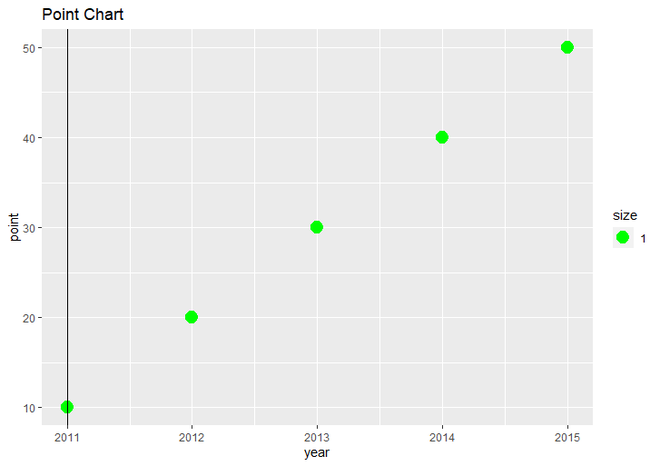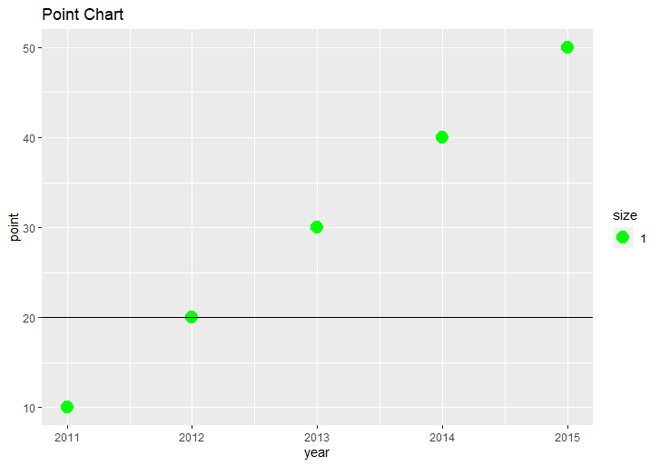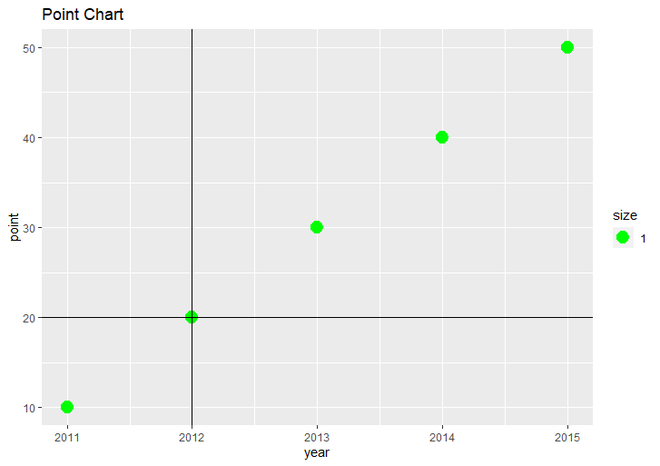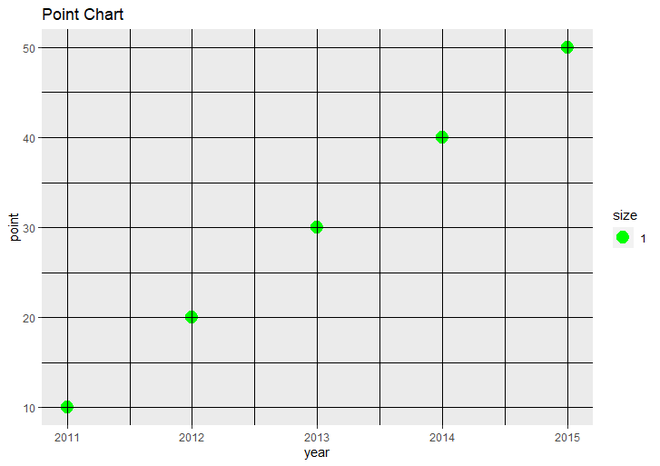# Add Vertical and Horizontal Lines to ggplot2 Plot in R

• Last Updated : 20 May, 2021

In this article, we will see how to add Vertical and Horizontal lines to the plot using ggplot2 in R Programming Language.

### Adding Vertical Line To R Plot using geom_vline()

For adding the Vertical line to the R plot, geom_vline() draws a vertical line at a specified position.

Syntax: geom_vline(xintercept)

Parameter: here xintercept is used control the X position of line.

Return: Vertical line on R plot.

Example: To add the vertical line on the plot, we simply add geom_vline() function to ggplot2() function and pass the xintercept, which basically has a location on X-axis, where we actually want to create a vertical line. Here we set 2011 to the xintercept.

## R

 `# load ggplot2 package` `library``(ggplot2)`   `# Create a dataframe for Plot data` `data <- ``data.frame``(year = ``c``(2011, 2012, 2013, 2014, 2015),` `                   ``point = ``c``(10, 20, 30, 40, 50))`   `# Plot the scatter plot with vertical line` `# at X=2011` `ggplot``(data, ``aes``(year, point)) +    ` `  ``ggtitle``(``"Point Chart"``)+` `  ``geom_point``(``aes``(size = 1.0), col = ``"green"``)+` `  ``geom_vline``(xintercept = 2011)`

Output:Scatter plot with vertical line at X=2011

### Adding Horizontal Line To R Plot using geom_hline()

And for adding Horizontal lines to the R plot, we will use geom_hline() function:

Syntax: geom_hline(yintercept)

Parameter: here yintercept is used control the y position of line.

Return: Horizontal line on R plot.

Example: To add the horizontal line on the plot, we simply add geom_hline() function to ggplot2() function and pass the yintercept, which basically has a location on the Y axis, where we actually want to create a vertical line. Here we set 20 to the xintercept.

## R

 `# load ggplot2 package` `library``(ggplot2)`   `# Create a dataframe for Plot data` `data <- ``data.frame``(year = ``c``(2011, 2012, 2013, 2014, 2015),` `                   ``point = ``c``(10, 20, 30, 40, 50))`   `# Plot the scatter plot with horizontal ` `# line at Y=20` `ggplot``(data, ``aes``(year, point)) +    ` `  ``ggtitle``(``"Point Chart"``)+` `  ``geom_point``(``aes``(size = 1.0), col = ``"green"``)+` `  ``geom_hline``(yintercept = 20)`

Output:Scatter plot with Horizontal line at Y=20

### Adding both vertical and horizontal lines to R plot

To add both lines, we add both geom_vline() and geom_hline() function to ggplot() function and set the values of xintercept and yintercept.

Example: Here we will draw the vertical line at X=2012 and the vertical line at Y=20.

## R

 `# load ggplot2 package` `library``(ggplot2)`   `# Create a dataframe for Plot data` `data <- ``data.frame``(year = ``c``(2011, 2012, 2013, 2014, 2015),` `                   ``point = ``c``(10, 20, 30, 40, 50))`   `# Plot the scatter plot with both lines` `# at X=2012 and Y=20` `ggplot``(data, ``aes``(year, point)) +    ` `  ``ggtitle``(``"Point Chart"``)+` `  ``geom_point``(``aes``(size = 1.0), col = ``"green"``)+` `  ``geom_vline``(xintercept = 2012)+` `  ``geom_hline``(yintercept = 20)`

Output:Scatter plot with both lines at X=2012 and Y=20

### Adding multiple vertical and horizontal lines to R plot using seq() function

We will use seq() function to represent the xintercept and yintercept and all other settings will remain the same as example 3.

Syntax: seq(from, to, by)

Parameters:

• from : it is used to represent starting length.
• to:  it is used to represent ending length.
• by: it represents increment of the system.

Return: well-formed sequence.

Example: Here we’re drawing vertical lines from 2011 to 2015 with a difference of 0.5 and horizontal lines from 10 to 50 with a difference of 5.

## R

 `# load ggplot2 package` `library``(ggplot2)`   `# Create a dataframe for Plot data` `data <- ``data.frame``(year = ``c``(2011, 2012, 2013, 2014, 2015),` `                   ``point = ``c``(10, 20, 30, 40, 50))`   `# Plot the scatter plot with multiple ` `# vertical and horizontal lines` `ggplot``(data, ``aes``(year, point)) +    ` `  ``ggtitle``(``"Point Chart"``)+` `  ``geom_point``(``aes``(size = 1.0), col = ``"green"``)+` `  ``geom_vline``(xintercept = ``seq``(from=2011, to=2015, by = 0.5))+` `  ``geom_hline``(yintercept = ``seq``(from=10, to=50, by = 5))`

Output:Scatter plot with multiple vertical and horizontal lines

My Personal Notes arrow_drop_up
Recommended Articles
Page :